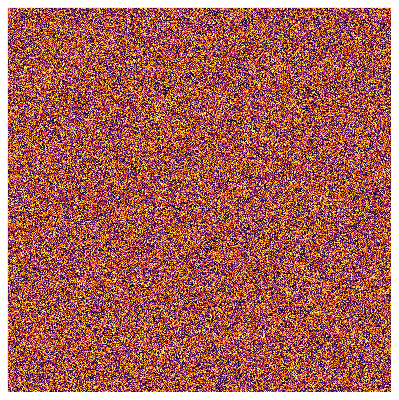# Plotting only the contents of a plot/heatmap and hiding everything else using Plots.jl

Hi! I would like to plot only the very contents of a plot (a heatmap in this case) using Plots.jl.

I’ve set the aspect ratio to 1:1, hidden the axes, hidden the color bar, set the margins to zero, and set the image size to match the size of the actual heatmap. However, I still get a white border around the plot, and the size of the plot is not exactly what I set it to be.

Here is a sample code:

``````using Plots
using Plots.PlotMeasures

const N = 400
const xs = 1:N
const ys = 1:N
const values = rand(N, N)

heatmap(
xs, ys, values,
aspect_ratio=:equal,  # 1:1 aspect ratio
axis=([], false),     # Hide axes
cbar=false,           # Hide color bar
margins=0px,          # Hide margins
size=(N, N),          # Figure size
)

savefig("plot.png")
``````Notice the white border around the plot and the `399 × 400` dimensions instead of `400 × 400`.

1 Like

There’s a built-in margin of 2 (mm?) with GR. (To be fixed… someday.) Try using a negative margin.